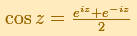The real cosine function can be extended using the exponential function:As a power series the equivalent definition is:This series converges everywhere in the complex plane.

If we increase the degree of the Taylor polynomial, this polynomial approaches the function more and more. We can see it if we look at the Remainder (the difference between the function and the polynomial).

For example, this is a representation of a Taylor's polynomial of degree 5 (In the mathlet we can change the center):And this is the remainder. We can see how the approximation is much better near the center:If we increase the degree of the polynomial the approximation is better (degree 10):The area where the approximation is good is much bigger:The Cosine Function is periodic with period.REFERENCES

Tristan Needham - Visual Complex Analysis. (pags. 84) - Oxford University PressThe Complex Cosine Function extends the Real Cosine Function to the complex plane. It is a periodic function that shares several properties with his real ancestor.The Complex Cosine Function maps horizontal lines to confocal ellipses.By increasing the degree, Taylor polynomial approximates the sine function more and more.The complex exponential function is periodic. His power series converges everywhere in the complex plane.By increasing the degree, Taylor polynomial approximates the exponential function more and more.We will see how Taylor polynomials approximate the function inside its circle of convergence.Examples of complex functions: polynomials, rationals and Moebius Transformations.